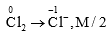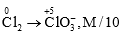When Cl2 is converted into Cl- & Cl${\mathrm{O}}_{3}^{-}$ then n-factor of Clwill be:

1. 3/5

2. 5/3

3. 7/2

4. 2

(2) Cl$\to$Cl-+ Cl${\mathrm{O}}_{3}^{-}$ (disproportionation)Eq. wt. of Cl$\frac{M}{2}$+$\frac{M}{10}$=$\frac{3M}{5}$

Hence, n-factor of Clwill be 5/3.

Difficulty Level:

• 13%
• 59%
• 17%
• 12%